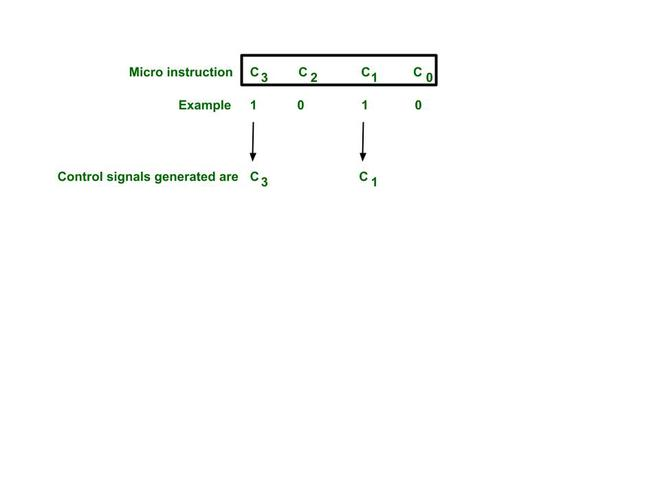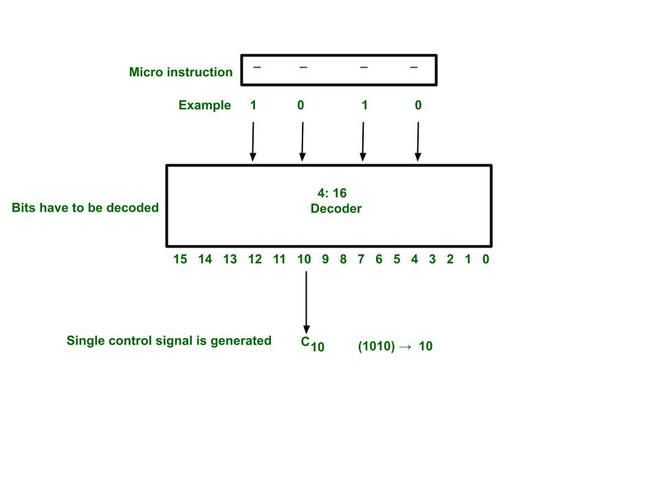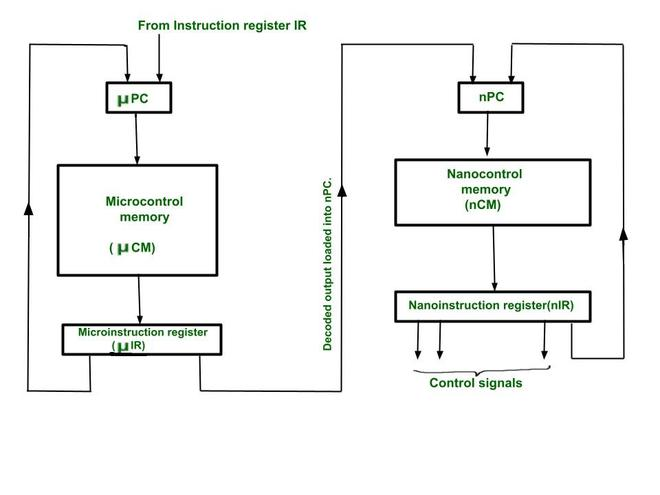# Nano programming in Micro Instruction Format

• Difficulty Level : Hard
• Last Updated : 22 Jul, 2021

In this article, we will discuss Nano programming in micro-instruction format in detail and also will understand with the help of example and also will discuss the need for Nano programming. Let’s discuss it one by one.

Pre-requisites –Horizontal and vertical microinstruction

Micro instruction format :
The control field of the micro-instruction decides the control signals to be produced. It is of two different formats Horizontal or Vertical. Let’s discuss it one by one.

Type-1 :
Horizontal micro-instruction :
Here, we will discuss the horizontal micro-instruction format as follows.

• Every bit of the micro-instruction corresponds to a control signal.
• The bit which is 1, that corresponding control signal will be produced by the micro-instruction. If there are N bits in micro-instruction then it can produce N control signals by that micro-instruction.
• As the control signals increase, the micro-instruction grows wider. Therefore, the control memory grows horizontally.
• Executes faster because no decoder is used.
• The control memory is large because the micro-instructions are wide.Horizontal micro-instruction format

Type-2 :
Vertical micro-instruction :
Here, we will discuss the vertical micro-instruction format as follows.

• The bits of the micro-instruction are decoded.
• The decoded output decides the control signal to be produced.
• N bits in the micro-instruction will totally generate 2N control signals.
• But one control can be generated by one micro-instruction.
• More micro-instructions are needed.
• Also, the decoding makes the execution slower.
• The circuit is complex.Vertical micro-instruction format

Need for nano programming :

• Horizontal micro-instructions can produce multiple control signals simultaneously but are very wide. This makes the control memory very large.
• Vertical micro-instructions are narrow, but after decoding, only produce one control signal. This makes the control memory small, but the execution is slow.
• Both methods have their own pros and cons.
• Hence, a combination of both techniques is needed to be called nano programming.

Nano programming :

1. Here we have a two-level control memory.
2. The instruction is fetched from the main memory into instruction register IR.
3. Using its opcode we load address of its first micro-instruction into µPC,
4. Using this address we fetch the micro-instruction from micro control memory (µCM) into micro instruction register µIR.
5. This is in vertical form and decoded by a decoder.
6. The decoded output loads a new address in a nano program counter (nPC).
7. By using this address, the nano-instruction is fetched from nano-control memory (nCM) into nano instruction register (nIR).
8. This is in horizontal form and can directly generate control signals which can be multiple at a time.
9. Such a combination gives advantage of both techniques.
10. The size of the control Memory is small as micro-instructions are vertical.Nano programming circuit.

Example –

• Suppose processors have 32 instructions (8 for arithmetic operations 8 for data transfer operations, 8 for logical operations 8 for shift operation). Suppose we require 32 control signals total in which 8 are responsible for performing data transfer operation, 8 for logical,8 for a shift, and 8 for arithmetic.
• If we implement it by horizontal micro-instruction, then the size of each micro-instruction should be 32 bits. Most probably for data transfer operation we don’t need a control signal for the other 3 types of instruction so in the whole micro-instruction the 27 bits for generating control signals for different 3 instruction types are 0 (garbage) which makes lots of memory space waste, also control memory size increases.
• If we use nano programming, then micro-control memory has instructions for identifying the type of the instruction by decoding i.e using vertical micro-instruction. After identifying the type of instruction the nano control memory is responsible for generating control signals for that particular instruction type that is identified previously. So the advantage of that is every nano instruction size reduces to 8 bits and every micro-instruction size reduces to 2 bits(for identifying 4 different types of instruction and for 2×4 decoder) i.e advantage of vertical micro-instruction also we can produce multiple control signal for a particular instruction type simultaneously which is an advantage of horizontal instruction.

#### Disadvantage of Nano programming

Increased memory access time:- The main disadvantage of the two level memory approaches is the loss of speed due to theextra memory access required for Nano control memory.

My Personal Notes arrow_drop_up